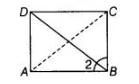# ABCD is a rectangle in which diagonal`
Question:

ABCD is a rectangle in which diagonal BD bisects ∠B. Show that ABCD is a square.

Solution:

Given In a rectangle $A B C D$, diagonal $B D$ bisects $\angle B$.

Construct Join AC.

To show $A B C D$ is a square.Proof

In $\triangle B A D$ and $\triangle B C D$, $\angle A B D=\angle C B D$ [given]

$\angle A=\angle C$ $\left[\right.$ each $\left.90^{\circ}\right]$

and $B D=B D$ [common side]

$\therefore \quad \triangle B A D \cong \triangle B C D \quad$ [by AAS congruence rule]

$\therefore \quad A B=B C$

and $A D=C D$ [by CPCT rule] ...(i)

But in rectangle $A B C D$, opposite sides are equal.

$\therefore \quad A B=C D$

and $B C=A D$ ...(ii)

From Eqs. (i) and (ii),

$A B=B C=C D=D A$

So, $A B C D$ is a square.

Hence proved.